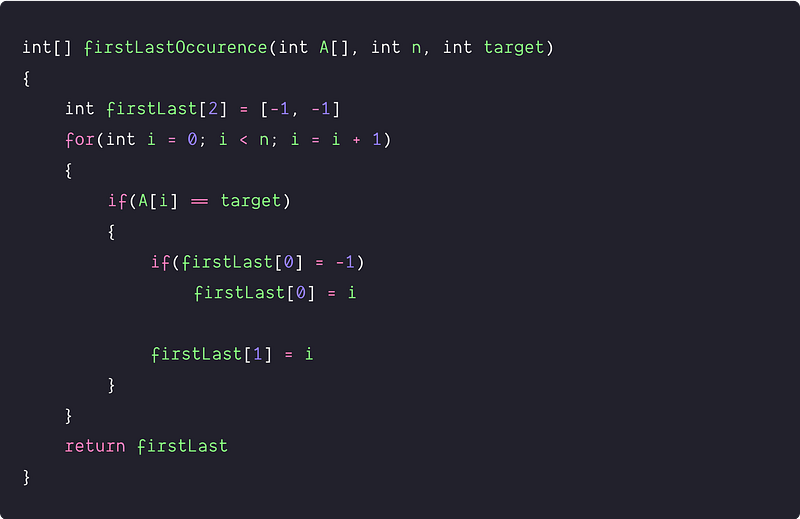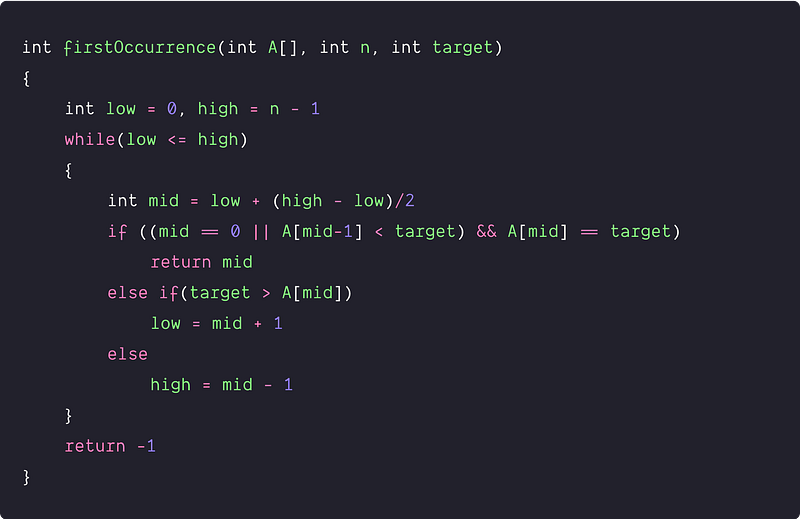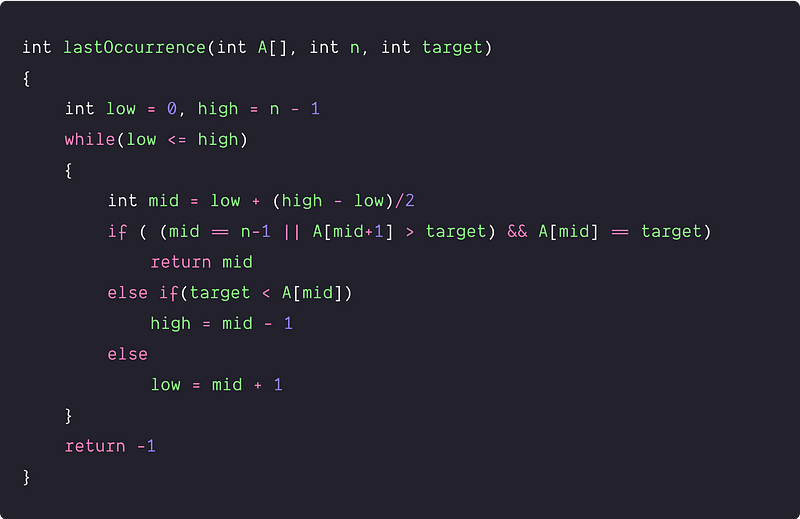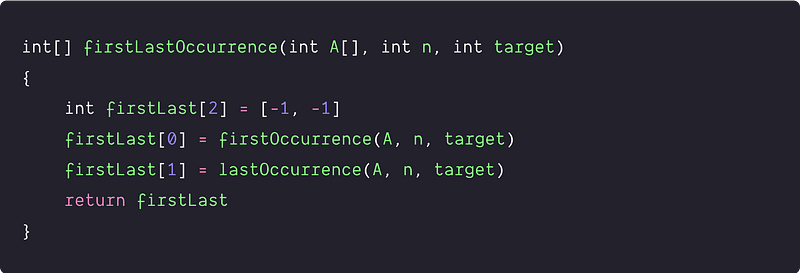# Find first and last positions of an element in a sorted array

Key takeaway: This is a good interview problem to learn problem-solving using binary search. We are applying binary search twice to improve the time complexity over the brute force approach.

### Let's understand the problem

Given an array of integers sorted in ascending order, write a code to find the first and last position of a given target value.

• If the target is not found in the array, return [-1, -1].
• We must design an algorithm with O(log n) time complexity.

Example 1

Input : A[] = [-1, 1, 2, 2, 2, 5 ,6, 12, 12], target = 2

Output : First Occurrence = 2, Last Occurrence = 4

Example 2

Input : A[] = [21, 32, 51, 70, 71], target = 70

Output : First Occurrence = 3, Last Occurrence = 3

### Discussed solution approaches

• A brute force approach  using linear search
• An efficient approach  by applying binary search twice

### A brute force approach using a linear search

Solution Idea

The straightforward approach is to traverse the array and track the index of the first and last occurrence of the target. This approach is inefficient because we did not take advantage of the fact that the given array is sorted.

Solution Steps

1. Take an extra constant size array firstLast to store the first and last occurrence of the target element. We will store the first occurrence at firstLast and the last occurrence at firstLast. At the start, we initialize both values with -1.
2. Now we traverse the array linearly from i = 0 to n-1 and update the firstLast when we encounter the target value for the first time. To keep track of the last occurrence, we keep updating the value of the firstLast every time we encounter the target value.
3. By the end of the loop, we return the firstLast[] array.

Solution PseudocodeTime and space complexity analysis

We are running a single loop n time and doing an O(1) operation at each iteration. So time complexity = n * O(1) = O(n). We are using constant extra space so that the space complexity would be O(1).

### An efficient approach using binary search

Solution Idea and Steps

Now critical questions are: can we improve the time complexity further? Can we use the sorted order property to enhance the efficiency of searching?

As we already know, searching any element in the sorted array, the idea of binary search works perfectly. It would take O(logn) time to search the target value in the sorted array. But the given problem is a little different from the binary search problem, where we need to search two occurrences of the target element. So how do we modify the standard binary search algorithm to solve it? Think.

The idea is simple: we can use binary search twice to solve the problem. The first binary search is to find the first occurrence of the target and the second binary search is to find the last occurrence of the target. Let’s design an algorithm for both the steps separately.

Binary search for finding the first occurrence

Let's use the iterative binary search! We first initialize the low = 0 and high = n -1 to track the left and right ends. Now we run a loop till low ≤ high:

• Calculate mid = low + (high-low)/2
• Now compare the value at mid with the target. The middle element will be the first occurrence in the two situations : 1) target == A[mid] and target > A[mid-1] i.e. when first occurrence is present somewhere in the middle 2) mid == 0 and A[mid] == target i.e. when the first occurrence is present at the first position. In both situations, we return mid-index as the first occurrence.

``````if ((mid == 0 || A[mid - 1] < target) && A[mid] == target)
return mid
``````
• If (target > A[mid]), then we need to search the first occurrence in the right part because all the value in the left part is less than the target. Update low = mid + 1
• But if both the above conditions are not satisfied, we need to search for the first occurrence in the left part (Think!). We update high = mid -1
• If we did not find the target value by the end of the loop, we return -1.

Pseudocode to find the first occurrenceBinary search for finding the last occurrence

Similarly, for finding the last occurrence, we also modify the binary search algorithm. We first initialize the low = 0 and high = n - 1 to track the left and right ends during the binary search loop. Now we run a loop till low ≤ high:

• Calculate mid = low + (high-low)/2
• Now compare the value at mid with the target. The mid element will be the last occurrence in the two situations: 1) target == A[mid] and target < A[mid + 1] i.e. when the last occurrence is present somewhere in the middle 2) mid == n-1 and A[mid] == target i.e. when the last occurrence is at the last position.
``````if ( (mid == n-1 || A[mid + 1] > target) && A[mid] == target)
return mid
``````
• If (target < A[mid]), then we need to search the last occurrence in the left part because all the value in the right part is less than the target. Update high= mid - 1
• But if both the above conditions are not satisfied, we need to search for the first occurrence in the right part (Think!). Update low = mid +1
• By the end of the loop, if we did not find the target value, return -1.

Pseudocode to find the last occurrenceSolution PseudocodeTime and space complexity analysis

Inside the function firstLastOccurrence(A[], n, target), we are doing some constant extra operations and applying binary search twice. So time complexity = O(1) + time complexity to search the first occurrence + time complexity to search the last occurrence = O(1) + O(logn) + O(logn) = O(log n).

We are using the implementation of iterative binary search, which takes O(1) extra space. So space complexity = O(1)

### Critical ideas to think!

• Can we solve this problem using a single binary search?
• How can we implement the above approach using a recursive binary search? What will be the space complexity?

### Comparisons of time and space complexities

• Using linear search: Time = O(n), Space = O(1)
• Using binary search: Time = O(logn), Space = O(1)

### Coding problems to practice using binary search

Thanks to Navtosh Kumar for his contribution in creating the first version of the content. Please write comments if you find an error or you want to share more insights about the topic. Enjoy learning, Enjoy coding, Enjoy algorithms!

Get well-designed application and interview centirc content on ds-algorithms, machine learning, system design and oops. Content will be delivered weekly.